# Distance Protection Working Principle & Fault Location Detection

## Distance Protection Relays Working Principle:

In last study we have discussed about only current or voltage based relay. Now we are going to discuss about current and voltage based relay. These relays are called as distance protection relays. The relay operation is purely depending upon the magnitude of the circuit current and voltage, typically the ratio of the circuit to be protected is calculated. The ratio of Voltage to current is called impedance. Here the prefix word distance mentions that impedance is nothing but an electrical measurement of distance along a transmission line. The relay measures the ratio of voltage and current. The ratio is less than a predetermined value, the relay trips the circuit breaker. The distance protection relay is also called as ratio relay.

Dependent on the ratio of V and I the distance relays are classified into three types

1. Impedance (Z) relay.
2. Reactance relay (X)
3. Admittance (Y) or Mho relay

### Impedance Relay

The impedance relay works based on the circuit quantities such as voltage and current. In this relay, there are two elements, the one produces a torque proportional to current while the other produces a torque proportional to voltage. The torque produced by the current element is equalized by the torque produced by the voltage element. The current element produces operating torque and voltage element produce the opposite torque to the current element. In other words, the torque produced by the voltage element is said to be negative torque.

Learn More:   Forward & Reverse Direction of Induction Motor and DC Motor

Voltage reference is taken from the potential transformer and the current reference is taken from the current transformer. The section AB of the line is protected zone which is impedance of line. Under normal conditions, the ratio of voltage V and current I is constant which is denoted as Z.  The relay is inoperative under this condition.

When the fault occurs at point X in the protected zone then the voltage drops while current increases. Thus the ratio of V/I. the impedance reduces. This is the impedance of line between relay located and the faulty point X. So when the impedance reduces than its predetermined value, the distance protection trips circuit breaker.

### Torque Equation

Consider The circuit current I, Voltage V, T operating torque. The positive torque is directly proportional to I2 and the negative torque is directly proportional to the voltage element V2.

Let control spring effect produces a constant torque of –K3, Hence the torque equation becomes,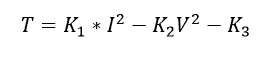where K1. K2, are the constants,

At the balance point, when the relay is on the verge of operating, the net torque is zero hence we can write,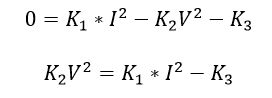Divide By K2 I2 on both sides..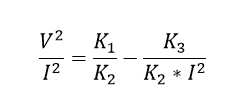As We Know The ratio of voltage to current is called impedance. It is denoted by Z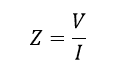Apply it on the above equation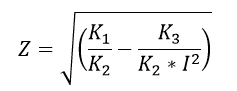Neglect the spring constant as it is absence in practical case. Hence K3=0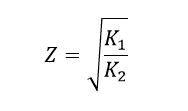Hence the ratio of V/I is constant at normal operating condition.

### How Distance Protection Detects the Fault location:

From the above V/I ratio, for the particular fault the impedance is constant. But The value of the ratio changes according to the fault location changes. Also the fault is nearer to relay, this ratio of the V/I will be low and as fault position moves away from the relay the ratio becomes higher and higher. So it can be installed to operate for particular location to be protected and After conducting relay adjustments for the particular location, it is inoperative beyond that section.